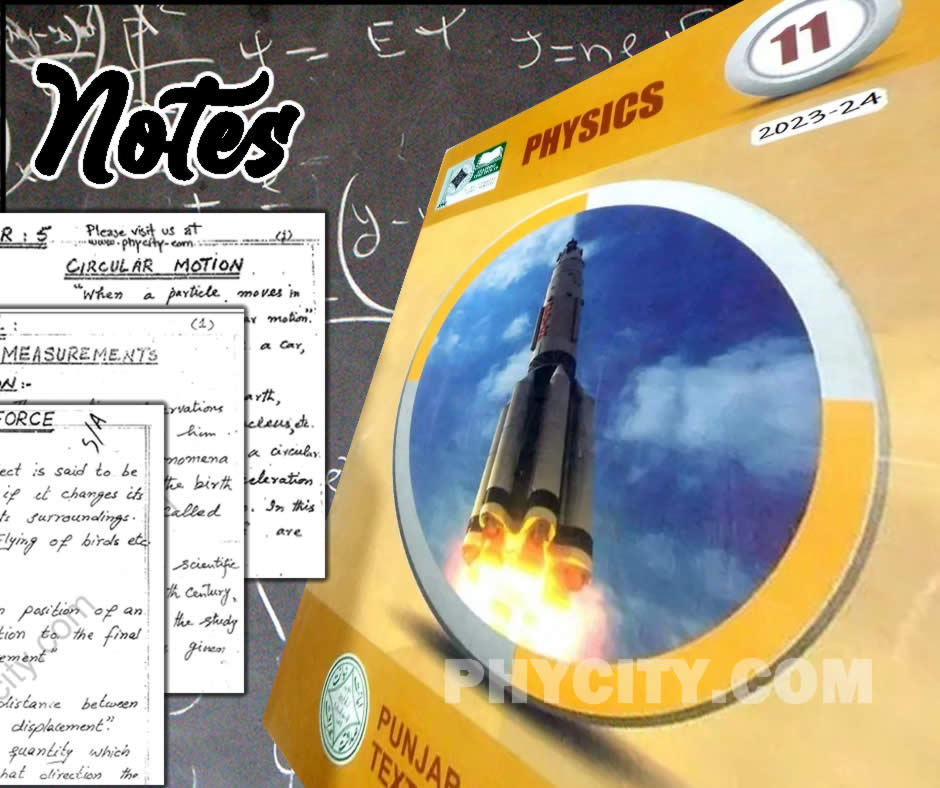fsc

# Notes of Physics 11 (PCTB)Physics 11 is published by Punjab Curriculum and Textbook Board (PCTB), Lahore, Pakistan. This book is the part of curricula of FSc Part 1 or HSSC 1.

There are 280 pages in the book consists of eleven chapters. The notes have been broken into three parts; theory, numerical and questions.

<columns 100% 50%>

Notes, answers to questions, solutions of numerical, model papers and old papers.

• Notes (Theory Notes, Solved Questions, Solved Numericals).

<newcolumn>

Notes, answers to questions, solutions of numerical, model papers and old papers.

• Notes (Theory Notes, Solved Questions, Solved Numericals).

</columns>

• fsc.txt